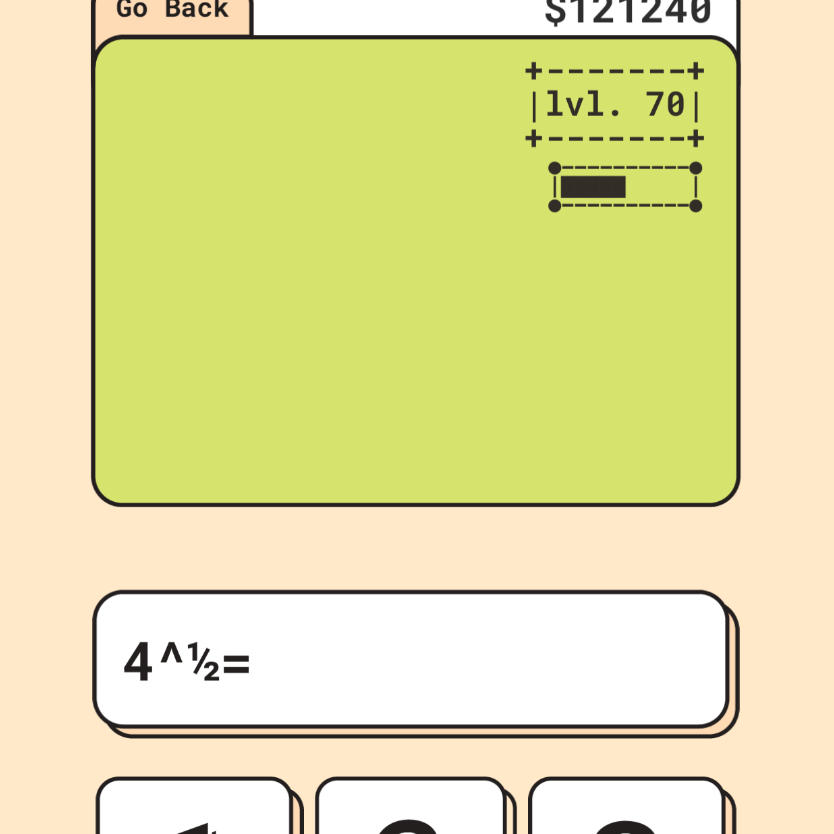## Jan 20th – Fixing & Balancing

After playtesting my game to get demo footage I realized that A) I get 0/1 quite a lot and B) The triple-digit problems get quite annoying and slow down the pacing of the game.

## TODAY’S OBJECTIVE:

1. Find why I am getting 0/1 = problems instead of actual division problems.
2. Adjust the problems given to either get rid of or limit the number of triple digit problems such as 934+196

After looking at the code and debugging I realized I had incorrect logic.

``````// old code
while ( ((int1 % int2) != 0) || (i < (max*100)) )
// new code
while ( ((int1 % int2) != 0) && (i < (max*100)) )``````

Because I was using the OR (||) operator the code would always run till the max interaction which does not make sense if I want it to stop when int1/int2 results in an integer. This was a quick fix as all I had to do was change it to be AND (&&) so when their was a remainder AND i is less than the max*100 keep generating new values. This means if their was no remainer I found a pair that results in integar division OR I have reached the max number of iterations.

To debug this code I used dotnetfiddle.net as the online IDE makes it quite easy to debug logic and simple problems instead of the unity console.

There were some other issues with another part of the division. The main thing was I was giving 0 for the denominator and so the remainder would always be 0 and therefore seem “okay” but result in failure because you cannot divide by 0.

I have been trying to think about how to fix this player experience issue for a long while. My first solution was to add radical problems. The reason why I did not do this is the only native vulgar fractions in superscript I could find were, ¼ ½ ¾ , so I could only really use ½ . Ideally I would also use one-third or cube root but I think I may just use the ½. I’ll be back…

After stumbling into bugs in the other part of the code I finally added the square root part. I took a more brutish approach using switch-cases to ensure that only perfect squares were served.

``````if(power == .5)
{
int randNum = UnityEngine.Random.Range(0, 13);
switch(randNum){ case 1: int1 = 1; break;
case 2: int1 = 4; break;
case 3: int1 = 9; break;
case 4: int1 = 16; break;
case 5: int1 = 25; break;
case 6: int1 = 36; break;
case 7: int1 = 49; break;
case 8: int1 = 64; break;
case 9: int1 = 81; break;
case 10: int1 = 100; break;
case 11: int1 = 121; break;
case 12: int1 = 144; break;}
} else {int1 = UnityEngine.Random.Range(min, max+1); }``````

Nonetheless, it works!

## NEXT OBJECTIVES

I think my main objective will be to set up the Algebra stage.

Share :